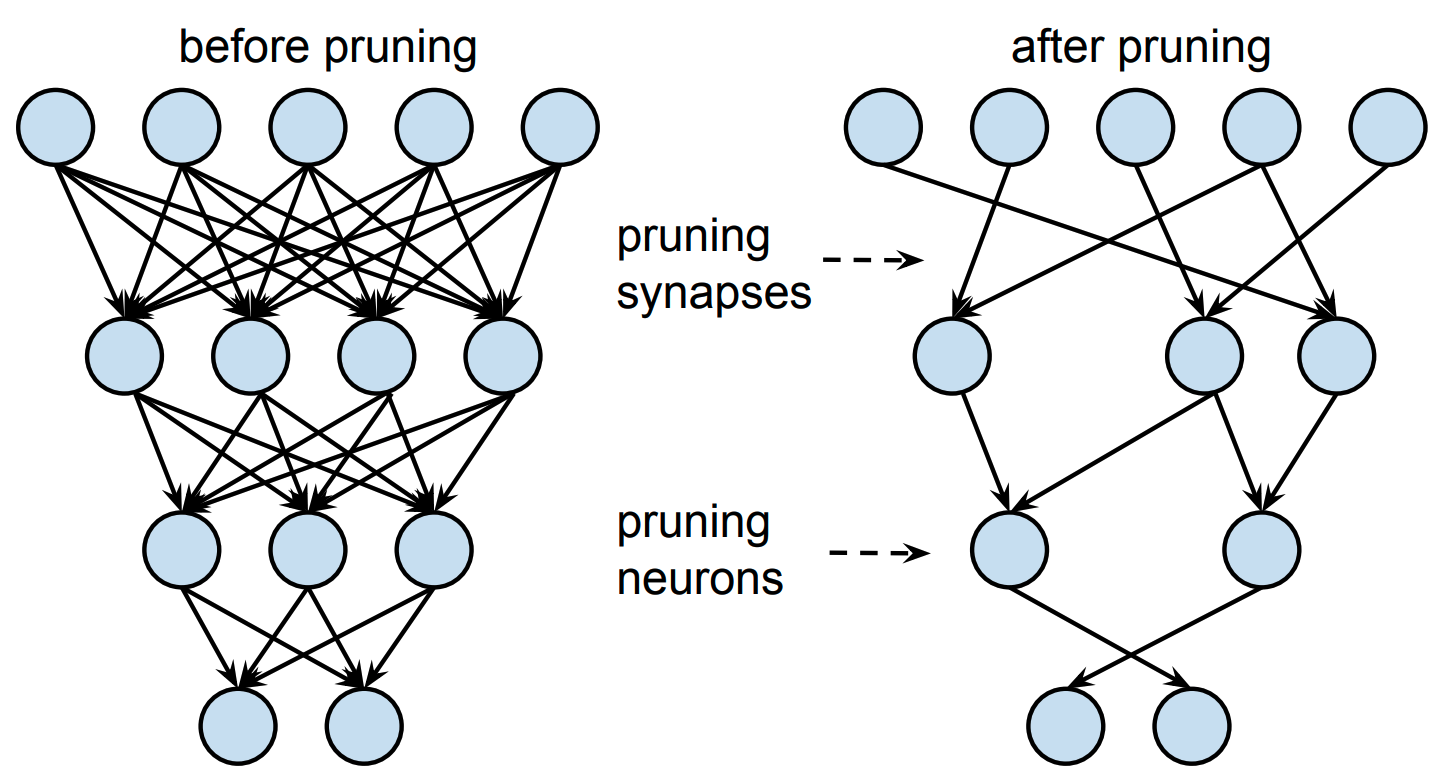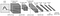# Experiments in Neural Network Pruning (in PyTorch).Photo by Noah Rosenfield on Unsplash

# Define pruningPruning synapses (=weights) vs pruning neurons (Taken from Learning both Weights and Connections for Efficient Neural Networks, 2015)

# II: Experiment SettingLeNet-5 architecture from Gradient-Based Learning Applied to Document Recognition (LeCun et al., 1998)
`class LeNet(nn.Module):    def __init__(self):        super().__init__()        self.conv1 = nn.Conv2d(1, 10, kernel_size=5)        self.conv2 = nn.Conv2d(10, 20, kernel_size=5)        self.conv2_drop = nn.Dropout2d()        self.fc1 = nn.Linear(320, 50)        self.fc2 = nn.Linear(50, 10)    def forward(self, x):        x = F.relu(F.max_pool2d(self.conv1(x), 2))        x = F.relu(F.max_pool2d(self.conv2_drop(self.conv2(x)), 2))        x = x.view(-1, 320)        x = F.relu(self.fc1(x))        x = F.dropout(x, training=self.training)        x = self.fc2(x)    return F.log_softmax(x, 1)`

## Pruning setup

`for i in 1 to K do    prune NN    finetune NN [for N epochs]end for`

Data Scientist